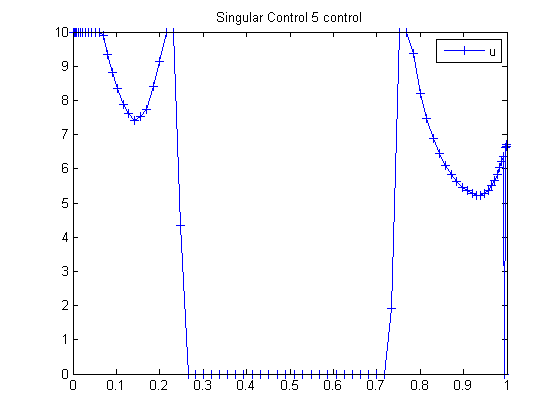105  Singular Control 5

ITERATIVE DYNAMIC PROGRAMMING, REIN LUUS

10.3 Yeo’s singular control problem

CHAPMAN & HALL/CRC Monographs and Surveys in Pure and Applied Mathematics

105.1  Problem Formulation

Find u over t in [0; 1 ] to minimize:

 J = x4(tF)

subject to:

 dx1 dt
= x2
 dx2 dt
= −x3*u + 16*x5 − 8
 dx3 dt
= u
 dx4 dt
= x12 + x22 + 0.0005*(x2+16*x5−8−0.1*x3*u2)2
 dx5 dt
= 1

The initial condition are:

 x(0) = [0  −1  −sqrt(5)  0  0]
 −4 <= u <= 10

The state x4 is implemented as a cost directly. x4 in the implementation is x5. u has a low limit of 9 in the code.

Reference: 

105.2  Problem setup

toms t
p = tomPhase('p', t, 0, 1, 80);
setPhase(p)

tomStates x1 x2 x3 x4
tomControls u

% Initial guess
x0 = {icollocate({x1 == 0; x2 == -1
x3 == -sqrt(5); x4 == 0})
collocate(u == 3)};

% Box constraints
cbox = {0 <= collocate(u) <= 10};

% Boundary constraints
cbnd = initial({x1 == 0; x2 == -1
x3 == -sqrt(5); x4 == 0});

% ODEs and path constraints
ceq = collocate({dot(x1) == x2
dot(x2) == -x3.*u + 16*x4 - 8
dot(x3) == u; dot(x4) == 1});

% Objective
objective = integrate(x1.^2 + x2.^2 + ...
0.0005*(x2+16*x4-8-0.1*x3.*u.^2).^2);

105.3  Solve the problem

options = struct;
options.name = 'Singular Control 5';
solution = ezsolve(objective, {cbox, cbnd, ceq}, x0, options);
t = subs(collocate(t),solution);
u = subs(collocate(u),solution);
Problem type appears to be: con
Starting numeric solver
===== * * * =================================================================== * * *
TOMLAB - Tomlab Optimization Inc. Development license  999001. Valid to 2011-02-05
=====================================================================================
Problem: ---  1: Singular Control 5             f_k       0.119318612949793070
sum(|constr|)      0.000000070783551966
f(x_k) + sum(|constr|)      0.119318683733345030
f(x_0)      1.024412849382205600

Solver: snopt.  EXIT=0.  INFORM=1.
SNOPT 7.2-5 NLP code
Optimality conditions satisfied

FuncEv  372 GradEv  370 ConstrEv  370 ConJacEv  370 Iter  346 MinorIter  939
CPU time: 10.265625 sec. Elapsed time: 10.609000 sec.

105.4  Plot result

figure(1)
plot(t,u,'+-');
legend('u');
title('Singular Control 5 control');